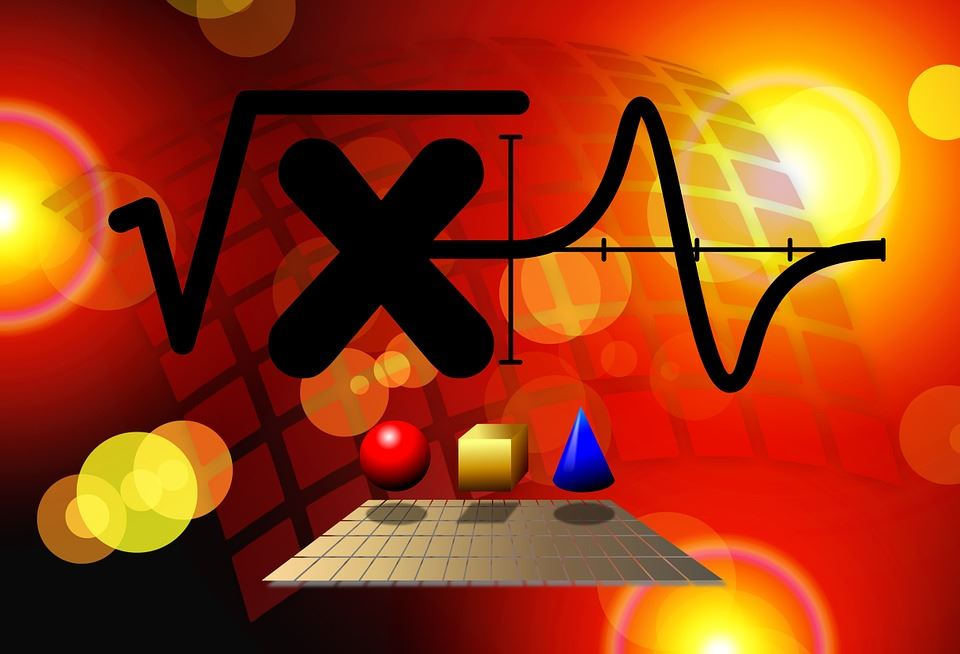# Branches Of Mathematics

Before the advent of the modern age, the study of mathematics was very limited. But over the period of time, mathematics has developed as a vastly diverse topic, and this is definitely not the limit. Developments still continue making large contributions to the field of technology. Hence, it is better termed as the Queen of Science. From the early number system to the modern research areas of computational sciences and probability, a number of new areas have evolved with the base of mathematics. With the expansion of the scope and usage of the subject, there is a corresponding need to classify different branches of mathematics.Branches of Mathematics

What are the Branches of Mathematics?

Mathematics can be broadly grouped into the following branches:

• Arithmetic: It is the oldest and the most elementary among other branches of mathematics. It deals with numbers and the basic operations- addition, subtraction, multiplication, and division, between them.
• Algebra: It is a kind of arithmetic where we use unknown quantities along with numbers. These unknown quantities are represented by letters of the English alphabet such as X, Y, A, B, etc. or symbols. The use of letters helps us to generalize the formulas and rules that you write and also helps you to find the unknown missing values in the algebraic expressions and equations.
• Geometry: It is the most practical branch of mathematics that deals with shapes and sizes of figures and their properties. The basic elements of geometry are points, lines, angles, surfaces, and solids.

There are some other branches of mathematics that you would deal with in the higher classes.

• Trigonometry: Derived from two Greek terms trignon (meaning a triangle) and metron (meaning a measure), it is the study of relationships between angles and sides of triangles.
• Analysis: It is the branch that deals with the study of the rate of change of different quantities. Calculus forms the base of analysis.

### What are the main branches of pure mathematics?

The main branches of pure mathematics are:

• Algebra
• Geometry
• Trigonometry
• Calculus
• Statistics and Probability

### What are the Branches of Mathematics for higher classes?

Mathematics in higher classes involves the following types:

• Analysis
• Discrete Maths
• Applied Mathematics
• Cartesian Geometry
• Matrix Algebra
• Combinatorics
• Topology
• Order theory

### What branch of mathematics is Calculus?

Calculus is one of the branches of mathematics which deals with the study of continuous change. The calculus mainly deals with the concepts of limits, derivatives, and integrals of various functions.